# Voltmeters and Multimeters

## Working Principle of Multimeter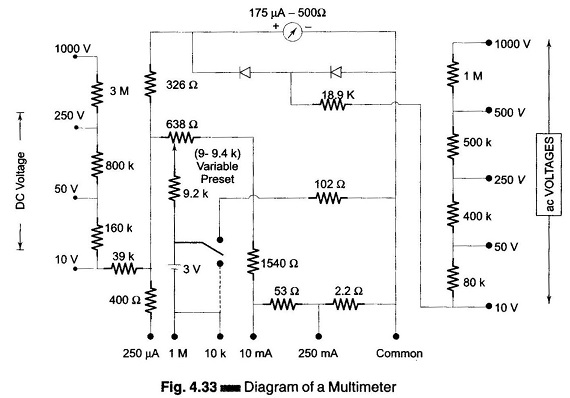Working Principle of Multimeter: A multimeter is basically a PMMC meter. To measure dc current the meter acts as an ammeter with a low series resistance. Range changing is accomplished by shunts in such a way that the current passing through the meter does not exceed the maximum rated value. A Working Principle of Multimeter …

## Considerations in Choosing an Analog Voltmeter

Considerations in Choosing an Analog Voltmeter: Considerations in Choosing an Analog Voltmeter has the following factors are to be considered. 1. Input Impedance The input impedance or resistance of the voltmeter should be as high as possible. It should always be higher than the impedance of the circuit under measurement to avoid the loading effect, …

## True RMS Voltmeter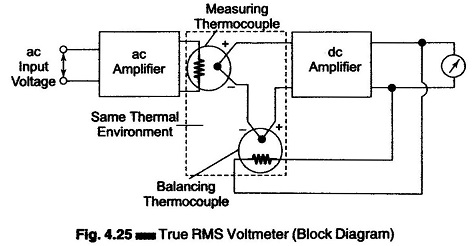True RMS Voltmeter: True RMS Voltmeter – Complex waveform are most accurately measured with an rms voltmeter. This instrument produces a meter indication by sensing waveform heating power, which is proportional to the square of the rms value of the voltage. This heating power can be measured by amplifying and feeding it to a thermocouple, …

## Average Responding Voltmeter and Peak Responding Voltmeter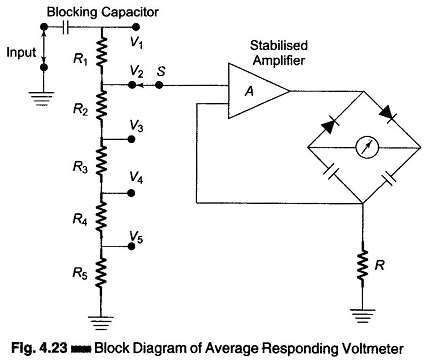Average Responding Voltmeter and Peak Responding Voltmeter: A simplified version of a circuit used in a typical average responding voltmeter is given in Fig. 4.23. The applied waveform is amplified in a high gain stabilized amplifier to a reasonably high level and then rectified and fed to a dc mA meter calibrated in terms of …

## AC Voltmeter using Half wave Rectifier | AC Voltmeter using Full wave Rectifier | Multirange AC Voltmeter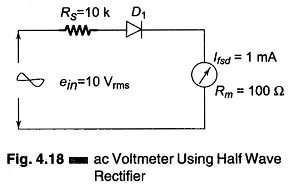AC Voltmeter using Half wave Rectifier | AC Voltmeter using Full wave Rectifier | Multirange AC Voltmeter: AC Voltmeter using Half wave Rectifier – If a diode D1 is added to the dc voltmeter, as shown in Fig. 4.18, we have an ac voltmeter using half wave rectifier circuit capable of measuring ac voltages. The …

## AC Voltmeter using RectifiersAC Voltmeter using Rectifiers: AC Voltmeter using Rectifiers – Rectifier type instruments generally use a PMMC movement along with a rectifier arrangement. Silicon diodes are preferred because of their low reverse current and high forward current ratings. Figure 4.16 (a) gives an ac voltmeter circuit consisting of a multiplier, a bridge rectifier and a PMMC …

## DC Standard Differential Voltmeter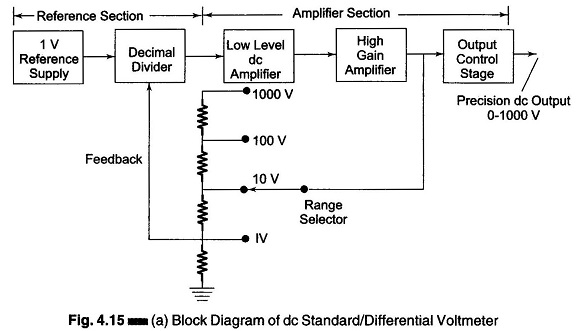DC Standard Differential Voltmeter: A basic DC Standard Differential Voltmeter can be operated in different modes. The three basic modes of operation are as a dc voltage standard, as a dc differential voltmeter, and as a dc voltmeter (conventional). 1. DC Voltage Standard: A + 1 V dc stable supply is obtained from a temperature …

## Differential Voltmeter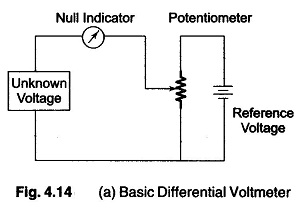Differential Voltmeter: The Differential Voltmeter technique, is one of the most common and accurate methods of measuring unknown voltages. In this technique, the voltmeter is used to indicate the difference between known and unknown voltages, i.e., an unknown voltage is compared to a known voltage. Figure 4.14 (a) shows a basic circuit of a differential …

Scroll to Top